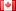# Revision history [back]

## Tried the following in sage. The answer is False, which is apparently incorrect. Anyone ever encountered this before? Any idea on what happened here?

forget() assume(x >= 0) expr = sin(x) < x

## Tried the following in sage. The answer is False, which is apparently incorrect. Anyone ever encountered this before? Any idea on what happened here?

forget()
assume(x >= 0)
expr = sin(x) < xx
print(bool(expr))


## Tried the following in sage. The answer is False, which is apparently incorrect. Anyone ever encountered this before? Any idea on what happened here?

forget()
assume(x >= 0)
expr = sin(x) < <= x
print(bool(expr))4 No.4 RevisionDSM5242 ●21 ●68 ●112

## Tried the following in sage. The answer is False, which is apparently incorrect. Anyone ever encountered this before? Any idea on what happened here?

forget()
assume(x >= 0)
expr = sin(x) <= x
print(bool(expr))